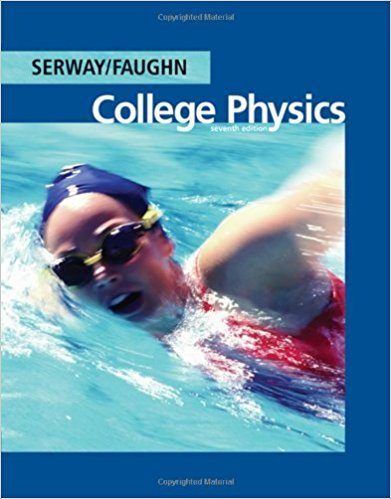×
Get Full Access to College Physics - 7 Edition - Chapter 14 - Problem 14.31
Get Full Access to College Physics - 7 Edition - Chapter 14 - Problem 14.31

×

# The ship in Figure P14.31 travels along a straight lineISBN: 9780495113690 154

## Solution for problem 14.31 Chapter 14

College Physics | 7th Edition

• Textbook Solutions
• 2901 Step-by-step solutions solved by professors and subject experts
• Get 24/7 help from StudySoup virtual teaching assistantsCollege Physics | 7th Edition

4 5 1 259 Reviews
27
5
Problem 14.31

The ship in Figure P14.31 travels along a straight line parallel to the shore and 600 m from it. The ships radio receives simultaneous signals of the same frequency from antennas A and B. The signals interfere constructively at point C, which is equidistant from A and B. The signal goes through the rst minimum at point D. Determine the wavelength of the radio waves.

Step-by-Step Solution:
Step 1 of 3

Chapter 31: Atomic Physics  Line spectrum of hydrogen: a series of individual lines caused by light being emitted by a low­pressure gas when subjected to a large electric field.  Wavelengths in the visible spectrum of hydrogen are given by the following equation: 1 =R 1 − 1 λ (2) n2 where n = 3, 4, 5,… and where R is the Rydberg constant 1.097 x 10^7 m^(­1)   Assumption of Bohr’s Model of the Hydrogen atom: o The electron in a hydrogen atom moves in a circular orbit around the nucleus o Only certain orbits are allowed, where the angular momentum in the nth allowed orbit is nh  L= 2π o Electrons in allowed orbits do not radiate. Radiation is emitted when an ele

Step 2 of 3

Step 3 of 3

##### ISBN: 9780495113690

Unlock Textbook Solution